Ex 8.1

Chapter 8 Class 10 Introduction to Trignometry (Term 1)
Serial order wise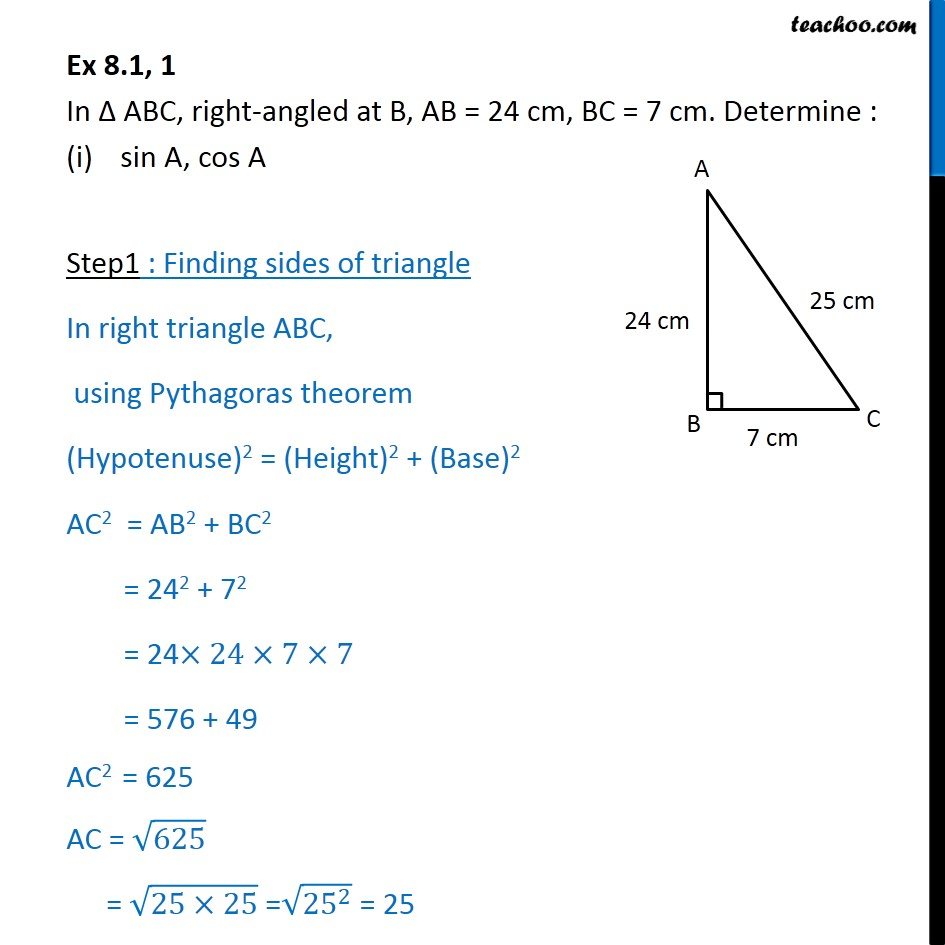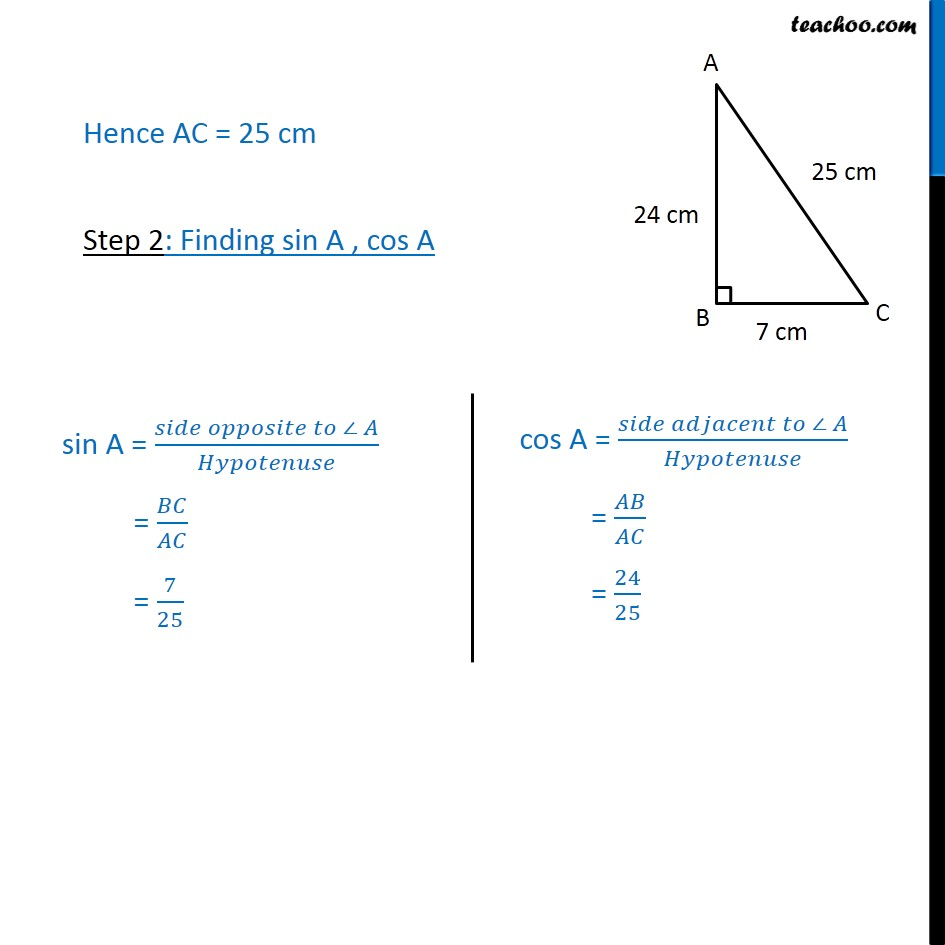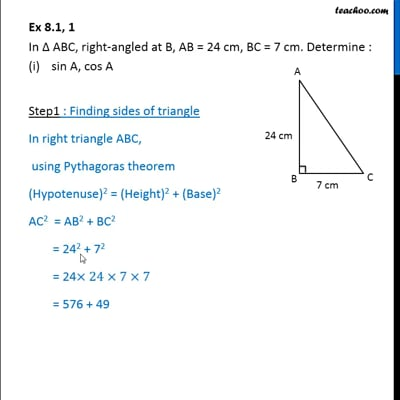This video is only available for Teachoo black users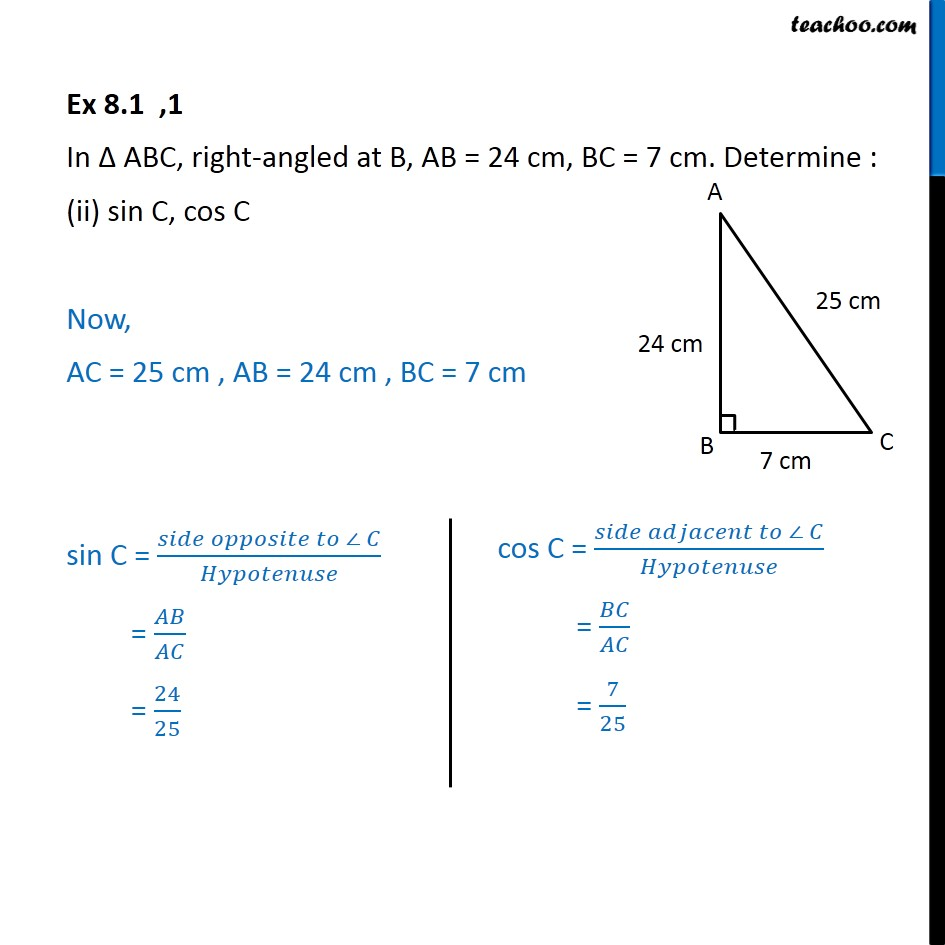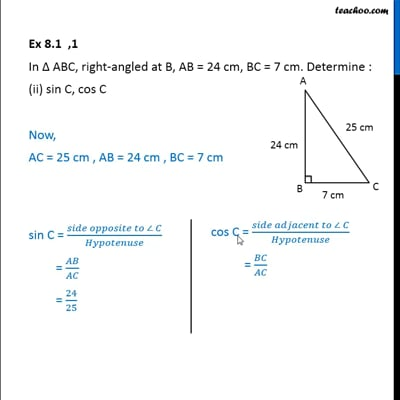This video is only available for Teachoo black users

### Transcript

Ex 8.1, 1 In Δ ABC, right-angled at B, AB = 24 cm, BC = 7 cm. Determine : sin A, cos A Step1 : Finding sides of triangle In right triangle ABC, using Pythagoras theorem (Hypotenuse)2 = (Height)2 + (Base)2 AC2 = AB2 + BC2 = 242 + 72 = 24×24×7×7 = 576 + 49 AC2 = 625 AC = √625 = √(25×25) =√(〖25〗^2 ) = 25 Hence AC = 25 cm Step 2: Finding sin A , cos A Ex 8.1 ,1 In Δ ABC, right-angled at B, AB = 24 cm, BC = 7 cm. Determine : (ii) sin C, cos C Now, AC = 25 cm , AB = 24 cm , BC = 7 cm sin C = (𝑠𝑖𝑑𝑒 𝑜𝑝𝑝𝑜𝑠𝑖𝑡𝑒 𝑡𝑜 ∠ 𝐶)/𝐻𝑦𝑝𝑜𝑡𝑒𝑛𝑢𝑠𝑒 = 𝐴𝐵/𝐴𝐶 = 24/25